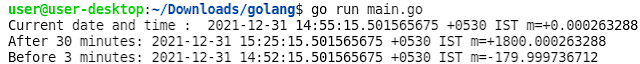## Posts

Showing posts with the label Time

### How to add/subtract Hour, Minute, Second, Millisecond, Microsecond and Nanosecond to a date in Go LanguageHello everyone, In this tutorial, we will learn h ow to add/subtract Hour, Minute, Second, Millisecond, Microsecond and Nanosecond to date in Go Language. The time package in Go language provides Add() function which  is used to add/subtract a duration to time t. Since duration can be represented in hours, minutes, seconds, milliseconds, microseconds and nanoseconds, The signature of the Add method is: func (t Time) Add(d Duration) Time Example 1: Go Program to add/subtract minutes. package main import ( "fmt" "time" ) func main() { //Current date and time dt1 := time.Now() fmt.Println( "Current date and time : " , dt1) //Adding 30 minutes dt2 := dt1.Add( 30 * time.Minute) fmt.Println( "After 30 minutes:" , dt2) //Subtract 3 minutes dt3 := dt1.Add(- 3 * time.Minute) fmt.Println( "Before 3 minutes:" , dt3) } Output: Example 2: Go Program to add/subtract seconds. package main import (